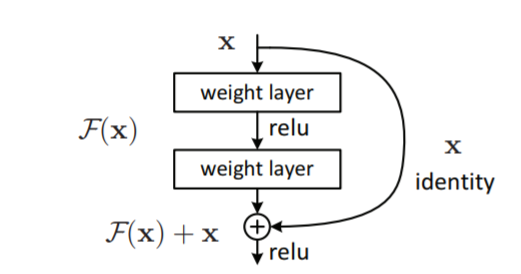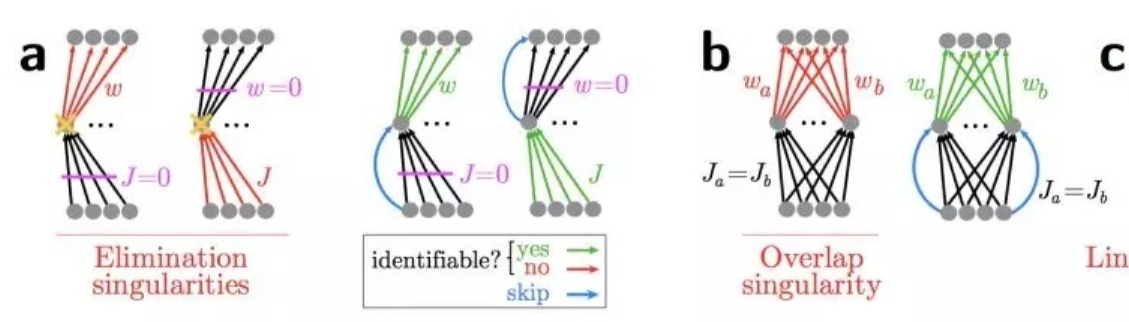对skip connect 和Identity Mapping的理解1、为什么使用skip connect

关于梯度问题

$$\begin{array}{c}{f^{\prime}=f\left(\boldsymbol{x}, \boldsymbol{w}_{\boldsymbol{f}}\right)} \\ {g^{\prime}=g\left(f^{\prime}\right)} \\ {y^{\prime}=k\left(g^{\prime}\right)} \\ {\text {cost}=\text {criterion}\left(y, y^{\prime}\right)}\end{array}$$

$$\frac{d\left(f^{\prime}\right)}{d\left(\boldsymbol{w}_{f}\right)} \times \frac{d\left(g^{\prime}\right)}{d\left(f^{\prime}\right)} \times \frac{d\left(y^{\prime}\right)}{d\left(g^{\prime}\right)} \times \frac{d(\cos t)}{y^{\prime}}$$

$$\frac{dh}{dx}=\frac{d\left(f+x\right)}{dx}=\frac{df}{dx}+1$$

模型退化2、Identity Mapping是什么\begin{aligned} \mathbf{y}_{l}=& h\left(\mathbf{x}_{l}\right)+\mathcal{F}\left(\mathbf{x}_{l}, \mathcal{W}_{l}\right) \\ & \mathbf{x}_{l+1}=f\left(\mathbf{y}_{l}\right) \end{aligned}

$$\mathbf{x}_{l+1}=\lambda_{l} \mathbf{x}_{l}+\mathcal{F}\left(\mathbf{x}_{l}, \mathcal{W}_{l}\right)$$

$$\mathbf{x}_{L}=\left(\prod_{i=l}^{L-1} \lambda_{i}\right) \mathbf{x}_{l}+\sum_{i=l}^{L-1} \hat{\mathcal{F}}\left(\mathbf{x}_{i}, \mathcal{W}_{i}\right)$$

$$\frac{\partial \mathcal{E}}{\partial \mathbf{x}_{l}}=\frac{\partial \mathcal{E}}{\partial \mathbf{x}_{L}}\left(\left(\prod_{i=l}^{L-1} \lambda_{i}\right)+\frac{\partial}{\partial \mathbf{x}_{l}} \sum_{i=l}^{L-1} \hat{\mathcal{F}}\left(\mathbf{x}_{i}, \mathcal{W}_{i}\right)\right)$$

Deep Residual Learning for Image Recognition

Highway Networks

Skip Connections Eliminate Singularities

Understanding and Improving Convolutional Neural Networks via Concatenated Rectified Linear Units

Highway and Residual Networks learn Unrolled Iterative Estimation

Residual Connections Encourage Iterative Inference

转载规则

《对skip connect 和Identity Mapping的理解》 ivory 采用 知识共享署名 4.0 国际许可协议 进行许可。
上一篇RefineNet Multi-Path Refinement Networks for High-Resolution Semantic Segmentation
RefineNet: Multi-Path Refinement Networks for High-Resolution Semantic Segmentation1.Abstract最近，很多CNN网络在物体检测领域有很出色的表现，甚至
2019-08-21源码编译pytorch及其编译知识

2019-07-31
目录